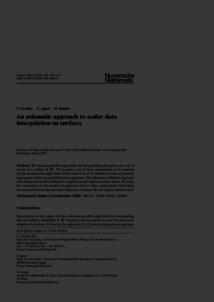Repository: Freie Universität Berlin, Math Department

# An axiomatic approach to scalar data interpolation on surfaces

Caselles, V. and Igual, L. and Sander, O. (2006) An axiomatic approach to scalar data interpolation on surfaces. Numerische Mathematik, 102 (3). pp. 383-411. ISSN 0029-599XPreview

386kB

Official URL: https://doi.org/10.1007/s00211-005-0656-8

## Abstract

We discuss possible algorithms for interpolating data given on a set of curves in a surface of ℝ^3. We propose a set of basic assumptions to be satisfied by the interpolation algorithms which lead to a set of models in terms of possibly degenerate elliptic partial differential equations. The Absolutely Minimizing Lipschitz Extension model (AMLE) is singled out and studied in more detail. We study the correctness of our numerical approach and we show experiments illustrating the interpolation of data on some simple test surfaces like the sphere and the torus.

Item Type: Article Mathematical and Computer Sciences > Mathematics > Numerical Analysis Department of Mathematics and Computer Science > Institute of Mathematics 1827 Ekaterina Engel 30 Mar 2016 18:35 03 Mar 2017 14:42

Repository Staff Only: item control page